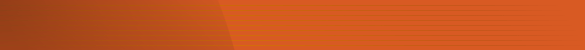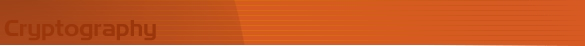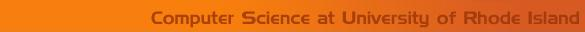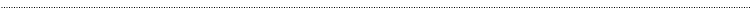Home Page Introduction Classical Ciphers Enigma Machine DES Key Agreement PGP R.S.A. Number Theory Other LinksClassical CryptographyVigenere Cipher Cryptanalysis Interactive Demo

STEP 1. INPUT PLAINTEXT AND CIPHERTEXT

The step shows letter frequencies for both plaintext and ciphertext. It also calculates the index of coincidence for the ciphertext and the estimated keyword length given by the Friedman test.

Ciphertext

RESULT OF STEP 1.

Table of Ciphertext letter frequencies.

 Letter Frequency Letter Frequency A N B O C P D Q E R F S G T H U I V J W K X L Y M Z

 The Index of Coincidence The estimated Keyword Length

STEP 2. ENTER KEYWORD SIZE

This step examines all cosets for keyword sizes 1,2,..,n. A statistic graph will be generated indicating the average of the incidence of coincidence for each cosets.

 Keyword Size

STEP 3. ENTER KEYWORD LENGTH

This step first determines the frequency of occurrence for each letter in the text. It then generates a statistic graph indicating the 26 dot products of the shifted frequency ratio vectors with the frequency vector for English.

 Keyword Length